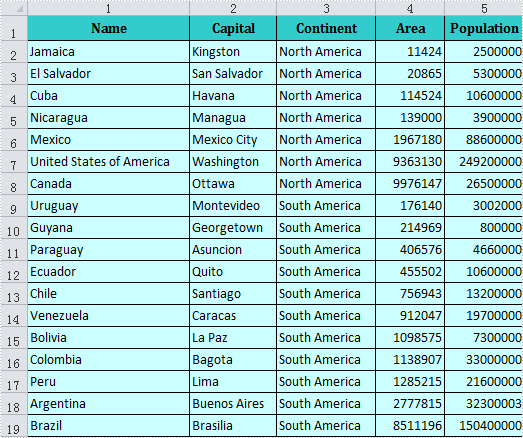# Set Excel Row Height and Column Width in C#, VB.NET

Mostly likely, we need to change the column width and row height when creating a spreadsheet with Microsoft Excel. Excel has enabled us to modify column width and row height in many different ways, such as dragging the boundary of a column or row to a desired size, inputting a certain value in the Column width box or Row height box. But for developers, how to achieve this purpose by programming? This article is aimed to delivering a simple solution by using Spire.XLS.

Look at the test file, in order to show complete data in the first column, we need to increase its width. And in order to emphasize item fields, we could add the height of the first row.

Test File:#### Code Snippet for Adjusting Row Height and Column Width

Step 1: Create a new instance of Workbook class and load the test file.

```Workbook workbook = new Workbook();
```

Step 2: Get the first worksheet from workbook.

```Worksheet sheet = workbook.Worksheets;
```

Step 3: Set the RowHeight=20 of the first row, set the ColumnWidth=23 of the first column.

```sheet.SetRowHeight(1, 20);
sheet.SetColumnWidth(1, 23);
```

Step 4: Save and launch the file.

```workbook.SaveToFile("Result.xls", ExcelVersion.Version97to2003);
System.Diagnostics.Process.Start(workbook.FileName);
```

Result:Full Code:

[C#]
```Workbook workbook = new Workbook();

Worksheet sheet = workbook.Worksheets;

sheet.SetRowHeight(1, 20);
sheet.SetColumnWidth(1, 23);

workbook.SaveToFile("Result.xls", ExcelVersion.Version97to2003);
System.Diagnostics.Process.Start(workbook.FileName);
```
[VB.NET]
```Dim workbook As New Workbook()